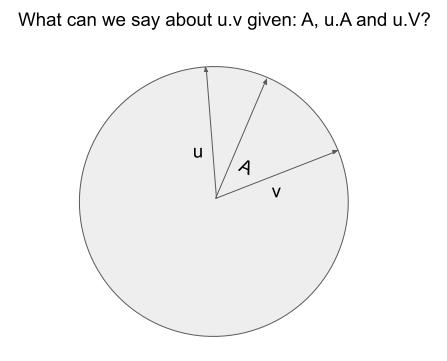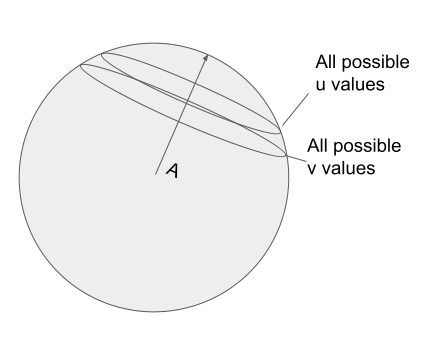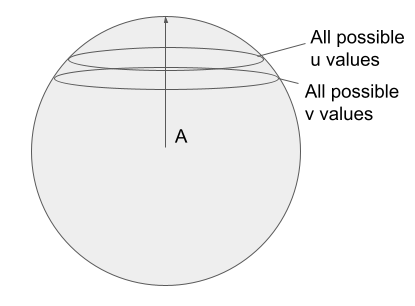Given three unit vectors u, v, and A - can we estimate the dot product of `u.v` if we only know `u.A` and `v.A`? Or put graphically:Well let’s take a stab at it. If we could solve this problem, it might have applications for a vector similarity system. We could have a shared reference point, A, store only A and the dot products to A, and use this to make estimates of their similarity to query vectors.

First step - if we don’t actually know `u` or `v`, only their dot products to A, to see all possible `u` and `v` values we need to think of them as more of a trace in the vector space. This trace corresponds to the set of all possible `u` or `v` that have our known `u.A`/`u.A`.We notice that as u.A and v.A both approach A, the circle shrinks in towards A, and its more likely that `u.v` is closer to 1.0.

We also notice that the traced circle is the root of the problem. Sometimes u and v might be on opposite sides of their traces. Sometimes they’re close together. Each possibility dictates a different `u.v`.

Luckily, as u and v converge towards A, the ‘wiggle room’ in these traces shrinks. As `u` and `v` converge towards A, we see `u.v` approach 1.0 - as we’d expect.

But how, mathematically, can we think about `u.v`?

We can rotate this sphere so that A becomes an axis. The 0th dimension corresponds to magnitude each vector has in the A’th dimension. In other words `v` and `u` becomes `v.A` and `u.A` respectively.0 1 n
u u.A ? ?
v u.A ? ?

Now what’s leftover - dimensions 1..n - are the trace we observe. We notice this is, itself, a sphere - and not a circle - at higher dimensions. I’ll just denote this leftover vector as `u1` and `v1`:

1 n
u1 ? ?
v1 ? ?

We can then breakdown `u.v` as:

`u.v = u.A * u.V + u1.v1`

We know from a previous post - `u1.v1` can be expected to be orthogonal at high dimensions - in other words 0 - which means `u.v` actually tends to approache `u.A * v.A`. Note this property remains true even though `u1` and `v1` are not unit vectors.

On the surface, this seems really useful! It’s also the case, however, that `u.A * v.A`, would also be expected to approach 0. Unless, of course, we’re cherry picking useful reference points, `A` in our dense vector space.

Of course, double check my math I could be wrong :).